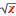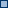# EditLive! 9 Documentation : Equation Editor Commands

In order for users to utilize the equation editing functionality, one of the following conditions must be met:

• Users are still operating the editor in their 30 day trial period.
• An Enterprise Edition license has been installed for the editor. For more information on licensing see the Licensing EditLive! article.

Function

XML Name Attribute

Shortcut

Image

Mnemonic

Insert a MathML mathematical equation.

InsertEquation

Insert Equation

N/AE

Edit a MathML mathematical equation

EditEquation...

Edit Equation...

N/A

N/A

N/A

## Attachments:equation_16.png (image/png)